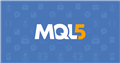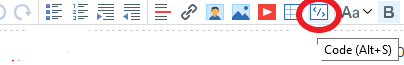# unexpected end of program

```#include <Expert\Expert.mqh>
#include <Expert\Signal\SignalFibonacci.mqh>
#include <Indicators\Indicators.mqh>
#include <Expert\Trailing\TrailingNone.mqh>
#include <Expert\Money\MoneyNone.mqh>

//+------------------------------------------------------------------+
//| Inputs |
//+------------------------------------------------------------------+
//--- inputs for expert
input string Inp_Expert_Title = "ExpertFibonacci";
int Inp_Expert_MagicNumber = 10981;
bool Inp_Expert_EveryTick = false;

//--- Input parameters for signal
input int Inp_Signal_Fibo_Period = 12;
input double Inp_Signal_Fibo_Level1 = 0.618;
input double Inp_Signal_Fibo_Level2 = 0.786;
input int Inp_Signal_Fibo_TakeProfit = 50;
input int Inp_Signal_Fibo_StopLoss = 20;
input bool Inp_Signal_Fibo_ConsiderDoji = true;

//--- Input parameters for Bollinger bands
input int Inp_BB_Period = 20;
input double Inp_BB_Deviation = 2.0;

//--- inputs for signal MACD
input int Inp_Signal_MACD_PeriodFast = 12;
input int Inp_Signal_MACD_PeriodSlow = 24;
input int Inp_Signal_MACD_PeriodSignal= 9;
input int Inp_Signal_MACD_TakeProfit = 50;
input int Inp_Signal_MACD_StopLoss = 20;

//+------------------------------------------------------------------+
//| Global expert object |
//+------------------------------------------------------------------+
CExpert ExtExpert;

//+------------------------------------------------------------------+
//| Initialization function of the expert |
//+------------------------------------------------------------------+
int OnInit(void)
{
//--- Initializing expert
if(!ExtExpert.Init(Symbol(), Period(), Inp_Expert_EveryTick, Inp_Expert_MagicNumber))
{
//--- failed
printf(FUNCTION + ": error initializing expert");
ExtExpert.Deinit();
return (-1);
}

//--- Creation of signal object
CSignalFibonacci* signal = new CSignalFibonacci;
if(signal == NULL)
{
//--- failed
printf(FUNCTION + ": error creating signal");
ExtExpert.Deinit();
return (-2);
}

//--- Add signal to expert (will be deleted automatically))
if(!ExtExpert.InitSignal(signal))
{
//--- failed
printf(FUNCTION + ": error initializing expert");
ExtExpert.Deinit();
return (-1);
```Documentation on MQL5: Language Basics / Preprocessor / Program Properties (#property)
• www.mql5.com
Program Properties (#property) - Preprocessor - Language Basics - MQL5 Reference - Reference on algorithmic/automated trading language for MetaTrader 5

You are missing a closing brace for the the OnInit() function, but there is a whole lot of missing code in general for it to compile correctly.

Also, please edit your post (don't create a new post) and replace your code properly (with "</>" or Alt-S), or attach the original file directly with the "+ Attach file" button below the text box.```THIS THE CODE /

include <Expert\Expert.mqh>
#include <Expert\Signal\SignalFibonacci.mqh>
#include <Indicators\Indicators.mqh>
#include <Expert\Trailing\TrailingNone.mqh>
#include <Expert\Money\MoneyNone.mqh>

//+------------------------------------------------------------------+
//| Inputs |
//+------------------------------------------------------------------+
//--- inputs for expert
input string Inp_Expert_Title = "ExpertFibonacci";
int Inp_Expert_MagicNumber = 10981;
bool Inp_Expert_EveryTick = false;

//--- Input parameters for signal
input int Inp_Signal_Fibo_Period = 12;
input double Inp_Signal_Fibo_Level1 = 0.618;
input double Inp_Signal_Fibo_Level2 = 0.786;
input int Inp_Signal_Fibo_TakeProfit = 50;
input int Inp_Signal_Fibo_StopLoss = 20;
input bool Inp_Signal_Fibo_ConsiderDoji = true;

//--- Input parameters for Bollinger bands
input int Inp_BB_Period = 20;
input double Inp_BB_Deviation = 2.0;

//--- inputs for signal MACD
input int Inp_Signal_MACD_PeriodFast = 12;
input int Inp_Signal_MACD_PeriodSlow = 24;
input int Inp_Signal_MACD_PeriodSignal = 9;
input int Inp_Signal_MACD_TakeProfit = 50;
input int Inp_Signal_MACD_StopLoss = 20;

//+------------------------------------------------------------------+
//| Global expert object |
//+------------------------------------------------------------------+
CExpert ExtExpert;

//+------------------------------------------------------------------+
//| Initialization function of the expert |
//+------------------------------------------------------------------+
int OnInit(void)
{
//--- Initializing expert
if (!ExtExpert.Init(Symbol(), Period(), Inp_Expert_EveryTick, Inp_Expert_MagicNumber))
{
//--- failed
printf(FUNCTION + ": error initializing expert");
ExtExpert.Deinit();
return (-1);
}

//--- Creating signal
CSignalFibonacci signal;
signal.Period(Inp_Signal_Fibo_Period);
signal.Level1(Inp_Signal_Fibo_Level1);
signal.Level2(Inp_Signal_Fibo_Level2);
signal.TakeLevel(Inp_Signal_Fibo_TakeProfit);
signal.StopLevel(Inp_Signal_Fibo_StopLoss);
signal.ConsiderDoji(Inp_Signal_Fibo_ConsiderDoji);
```

1. I stated ... edit your post (don't create a new post)
2. The code in your second post is missing even more code.
3. Just attach the file instead as it seems you are unable to paste it properly

Ian Emmanuel #:

that is the file i copied and pasted :

...Sergey Golubev #:

i just did it

Ian Emmanuel #:
i just did it

but i am putting this here too:

<improperly posted code deleted>

I give up!

Ian Emmanuel #: i just did it

You were asked to edit the original post and use the code button. You created a new one (#2).

Ian Emmanuel #: but i am putting this here too:
You were asked to edit the original post and use the code button. You created another new one (#6) and did not use the code button and did not delete the extra post.
Fernando Carreiro #: I give up!

I agree. Can not be helped. On my Do Not Help list.

Reason: